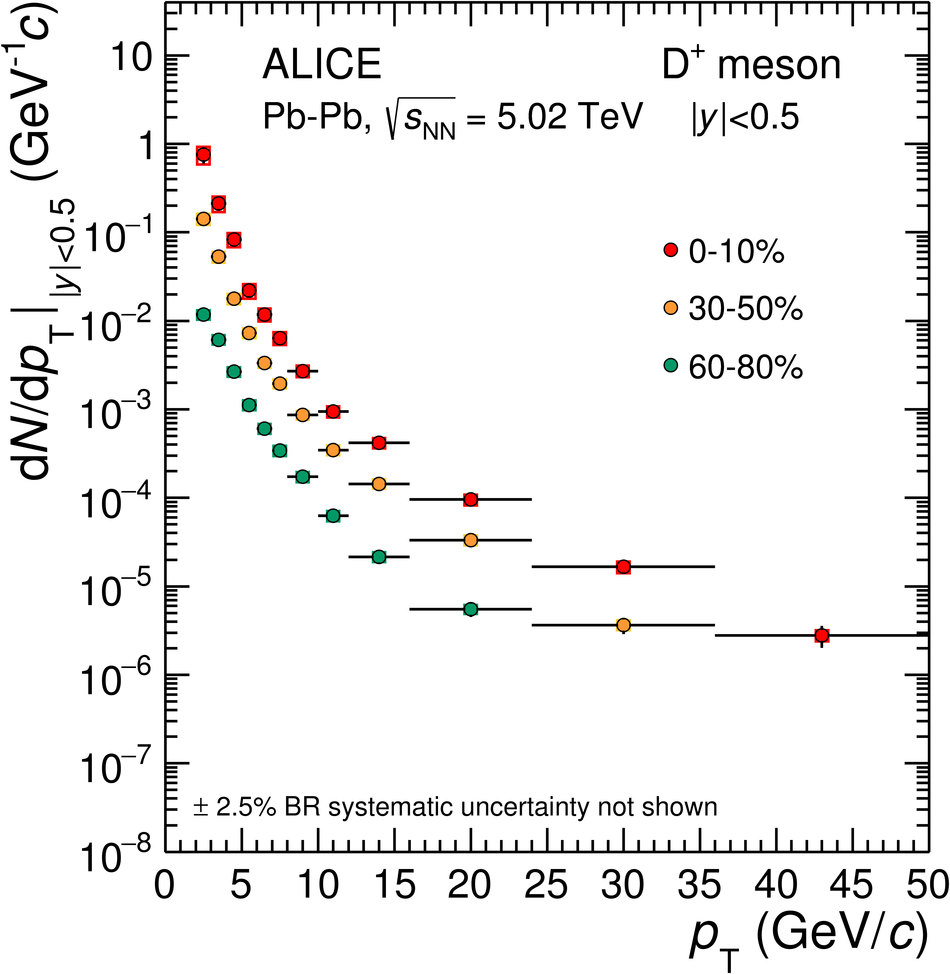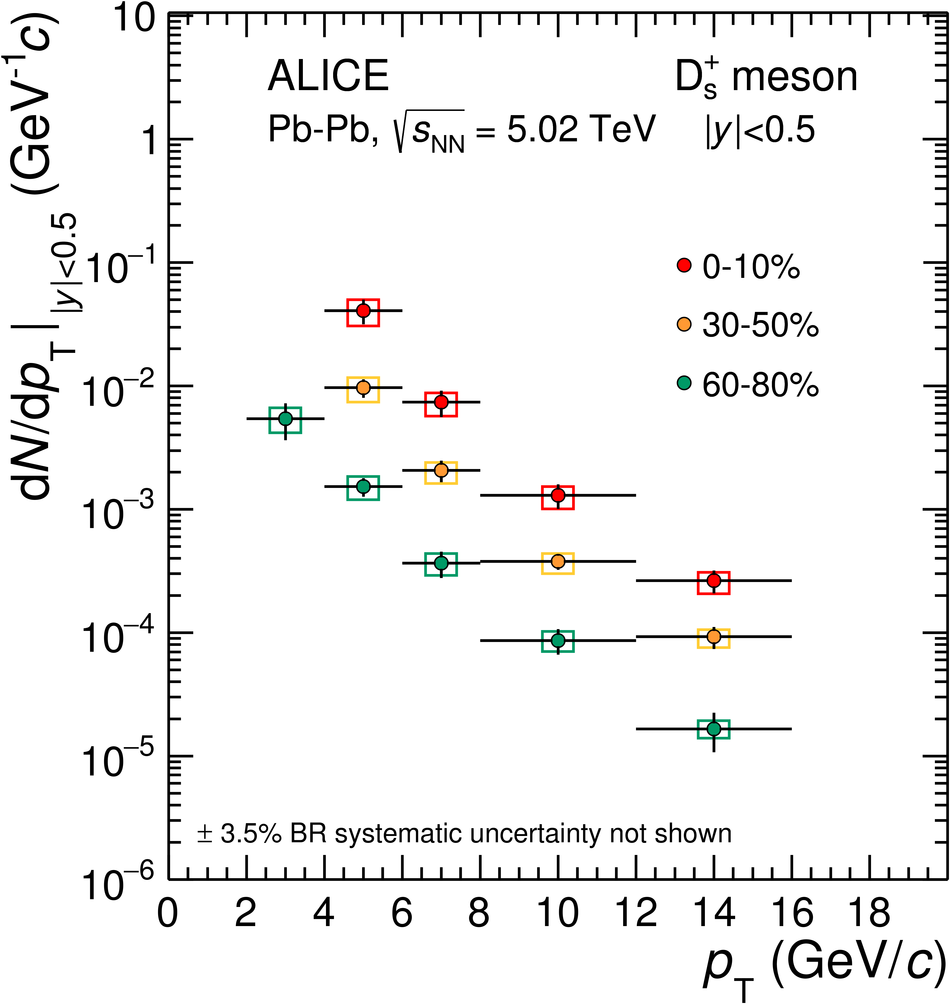# Figure 3

 Transverse momentum distributions $\d N/\d\pt$ of prompt $\Dzero$ (a), $\Dplus$ (b), $\Dstar$ (c) and $\Ds$ (d) mesons in the 0--10\%, 30--50\% and 60--80\% centrality classes in $\PbPb$ collisions at $\sqrtsNN=5.02~\tev$. Statistical uncertainties (bars) and systematic uncertainties (boxes) are shown. The uncertainty on the branching ratios is quoted separately Horizontal bars represent bin widths, symbols are placed at the centre of the bin.